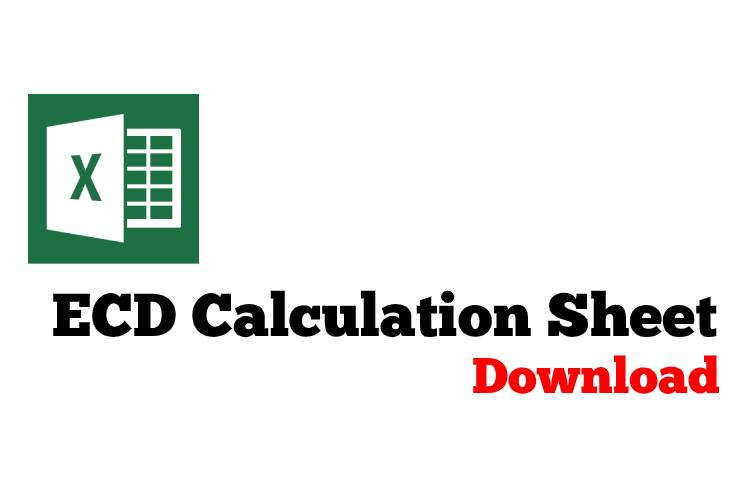# Equivalent Circulating Density (ECD) in both Oilfield and Metric Unit

Equivalent Circulating Density (ECD) is the effective density that combines current mud density and annular pressure drop. ECD is vital for drilling engineering since it limits how depth of each section to be drilled and leads to losses.The equivalent circulating density formula is shown below;

## Oilfield Unit

Equivalent Circulating Density (ECD) = (Annular Pressure Loss ÷ 0.052 ÷ True Vertical Depth (TVD)) + (Current Mud Weight)

Equivalent Circulating Density (ECD) in ppg

Annular Pressure Loss in psi

True Vertical Depth (TVD) in ft

Current Mud Weight in pppg

Example:

Annular pressure loss = 400 psi
True Vertical Depth = 8,000 ft
Current mud weight in ppg = 10 ppg

ECD in ppg =   (400 psi ÷ 0.052 ÷ 8,000 ft) +10.0 ppg

ECD = 11.0 ppg

## Metric Unit

Equivalent Circulating Density (ECD) = (Annular Pressure Loss × 1000 ÷ 9.81÷ True Vertical Depth (TVD) ) + (Current Mud Weight)

Equivalent Circulating Density (ECD) in kg/m3

Annular Pressure Loss in KPa

True Vertical Depth (TVD) in m

Current Mud Weight in kg/m3

Example:

Annular pressure loss = 2760 KPa
True Vertical Depth = 2,440 m
Current mud weight in ppg = 1200  kg/m3

ECD in ppg =  (2,760 Kpa  × 1000 ÷ 9.81÷ 2,440 m )  + 1,200 kg/m3

ECD = 1,315 kg/m3

Please find the Excel sheet to calculate Equivalent Circulating Density (ECD)Ref books:

Lapeyrouse, N.J., 2002. Formulas and calculations for drilling, production and workover, Boston: Gulf Professional publishing.

Bourgoyne, A.J.T., Chenevert , M.E. & Millheim, K.K., 1986. SPE Textbook Series, Volume 2: Applied Drilling Engineering, Society of Petroleum Engineers.

Mitchell, R.F., Miska, S. & Aadny, B.S., 2011. Fundamentals of drilling engineering, Richardson, TX: Society of Petroleum Engineer

Share the joyWorking in the oil field and loving to share knowledge.

### 14 Responses to Equivalent Circulating Density (ECD) in both Oilfield and Metric Unit

1.Andrew oloko says:

I love well control and answers.

2.Mahsun Hammedi says:

Why you only use annular pressure drop?
what about pressure loss inside the pipes?

•DrillingFormulas.Com says:

Mahsun,

The reason why using annular pressure drop is the direction of pressure drop acting downward which cause additional pressure to the bottom hole. The drill pipe pressure loss is acting upward therefore it cannot be used for ECD calculation.

Regards,
Shyne.

3.Francis Okoto says:

How can we use this during cement to calculate the ECD especially during cement displacement.

•DrillingFormulas.Com says:

If you want to use for cement displacement, you need to separate each section of fluid in the annuals in order to calculate annular pressure loss.

4.Egede Frank Chukwueku says:

plenty thanks to you all. its great to be part of this group

5.Arslan says:

Can we calculate the ECD if the surface pressure is not equal to zero? Or the surface pressure does not matter?

•DrillingFormulas.Com says:

Yes. You can add surface pressure into the calculation. This concepth is used in managed pressure while drilling.

6.Mide says:

Whoa I love this platform. Please I would like to know the relationship between ECD and EMW

This site uses Akismet to reduce spam. Learn how your comment data is processed.